# Bernoulli trials

Jump to: navigation, search

2010 Mathematics Subject Classification: Primary: 60-01 [MSN][ZBL]

Independent trials, each one of which can have only two results ( "success" or "failure" ) such that the probabilities of the results do not change from one trial to another. Bernoulli trials are one of the principal schemes considered in probability theory.

Letbe the probability of success, letbe the probability of failure, and let 1 denote the occurrence of success, while 0 denotes the occurrence of a failure. The probability of a given sequence of successful or unsuccessful events, e.g.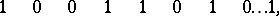is equal to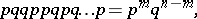whereis the number of successful events in the series oftrials under consideration. Many frequently occurring probability distributions are connected with Bernoulli trials. Let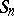be the random variable which is equal to the number of successes inBernoulli trials. The probability of the event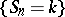is then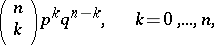i.e.has a binomial distribution. As, this distribution can be approximated by the normal distribution or by the Poisson distribution. Letbe the number of trials prior to the first success. The probability of the eventthen is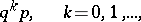i.e.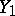has a geometric distribution. If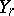is the number of failures which precede the-th appearance of a successful result,has the so-called negative binomial distribution. The number of successful outcomes of Bernoulli trials can be represented as the sumof independent random variables, in which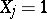if the-th trial was a success, and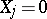otherwise. This is why many important laws of probability theory dealing with sums of independent variables were originally established for Bernoulli trial schemes (cf. Bernoulli theorem ((weak) Law of large numbers); Strong law of large numbers; Law of the iterated logarithm; Central limit theorem; etc.).

A rigorous study of infinite sequences of Bernoulli trials requires the introduction of a probability measure in the space of infinite sequences of zeros and ones. This may be done directly or by the method illustrated for the case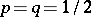below. Letbe a number, uniformly distributed on the segment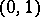, and letwhere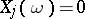or 1, be the expansion ofinto a binary fraction. Then the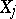,are independent and assume the values 0 and 1 with probability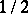each, i.e. the succession of zeros and ones in the expansion ofis described by the Bernoulli trial scheme with. However, the measure oncan also be specified so as to obtain Bernoulli trials with any desired(ifthe measure obtained is singular with respect to the Lebesgue measure).

Bernoulli trials are often treated geometrically (cf. Bernoulli random walk). Certain probabilities of a large number of events connected with Bernoulli trials were computed in the initial stage of development of probability theory in the context of the ruin problem.